## 软件工程第二次作业：熟悉使用工具 ——Author：Charlie

| GIT地址 |https://github.com/Charliewhy/Ilovecoding|
| ------ | ------ | ------ |
| GIT用户名| Charliewhy |
| 学号后5位 | 83104 |
|博客地址| https://www.cnblogs.com/CharlieWhy/ |
| 作业链接 | https://edu.cnblogs.com/campus/xnsy/Autumn2019SoftwareEngineeringFoundation/homework/7590 |

### 2.克隆项目 从班级要求仓库地址Fork到自己GitHub的仓库中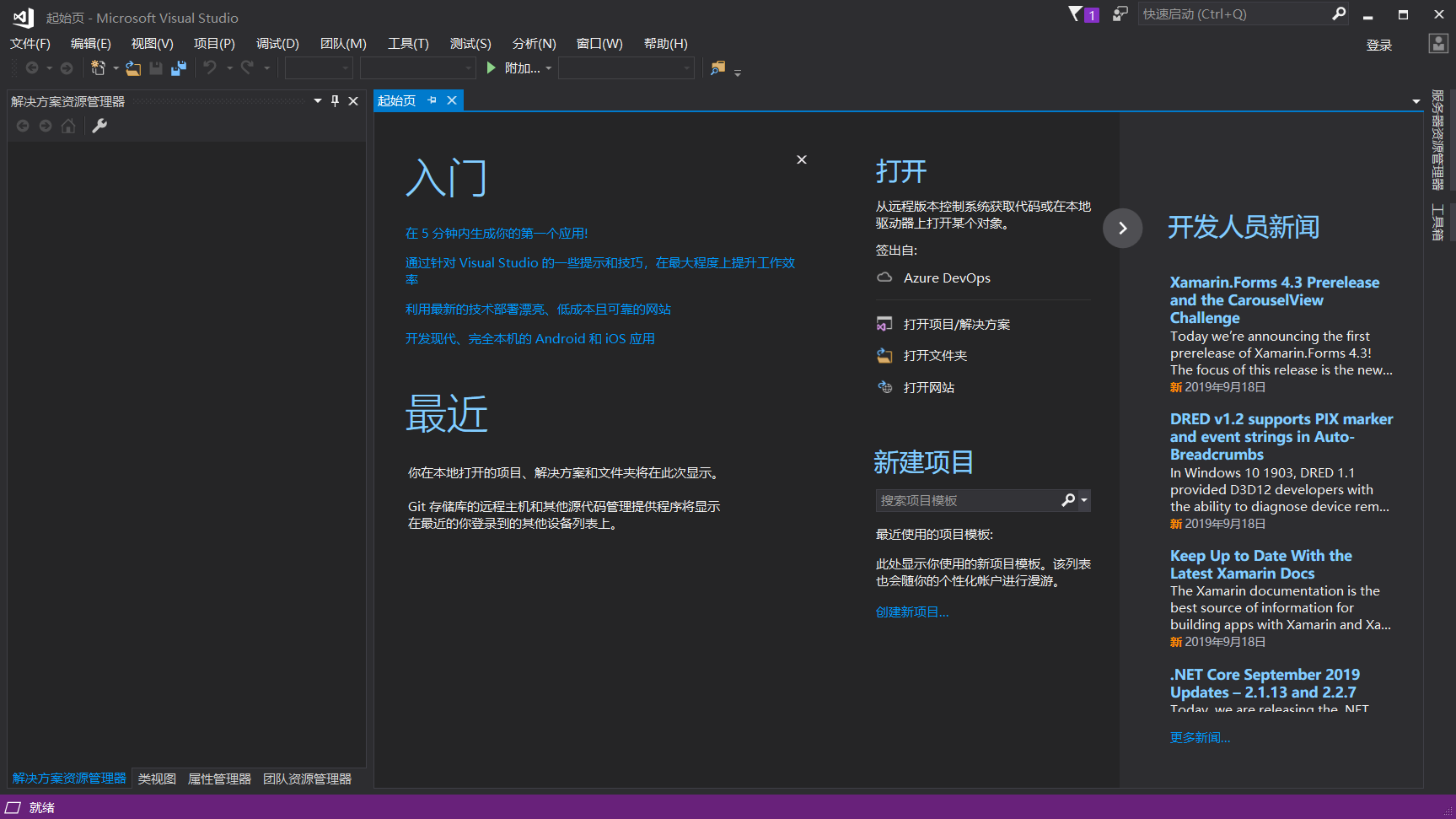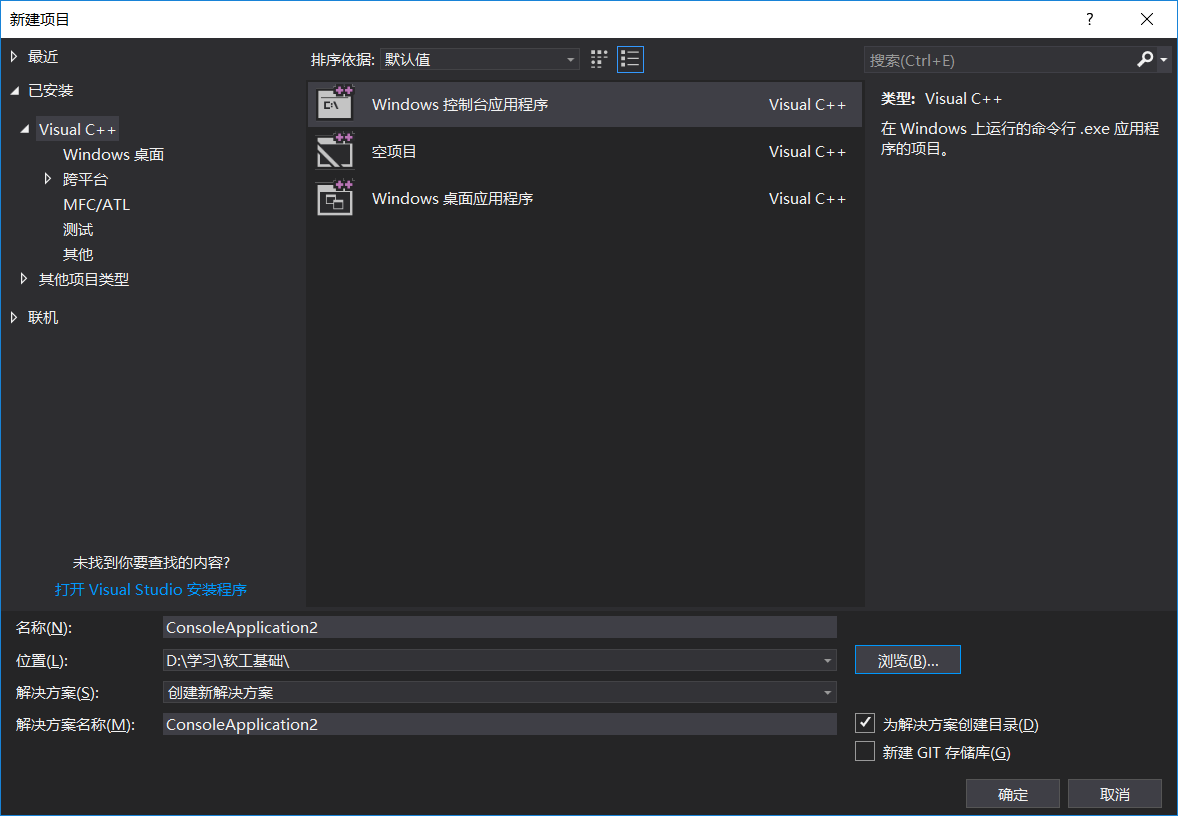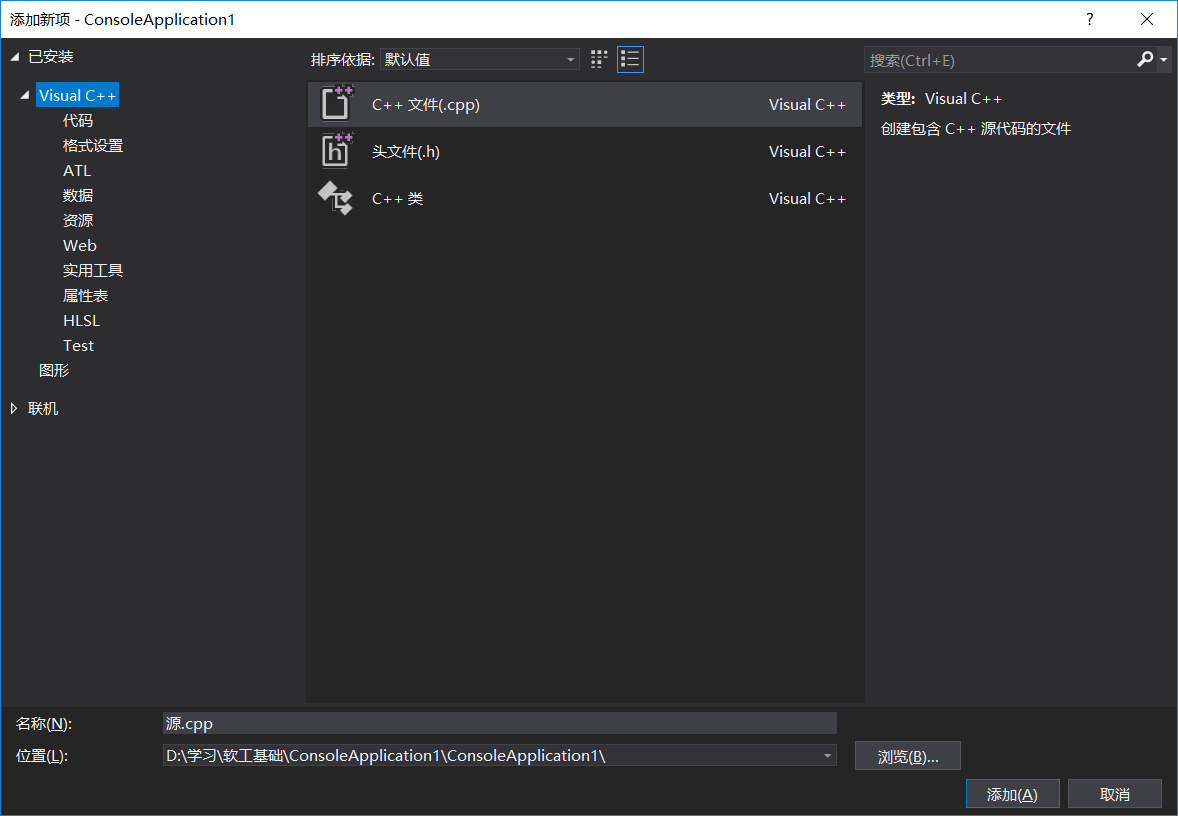#### 安装将仓库内容拷贝到本地的工具Git

Git-windows版本下载地址:点击此处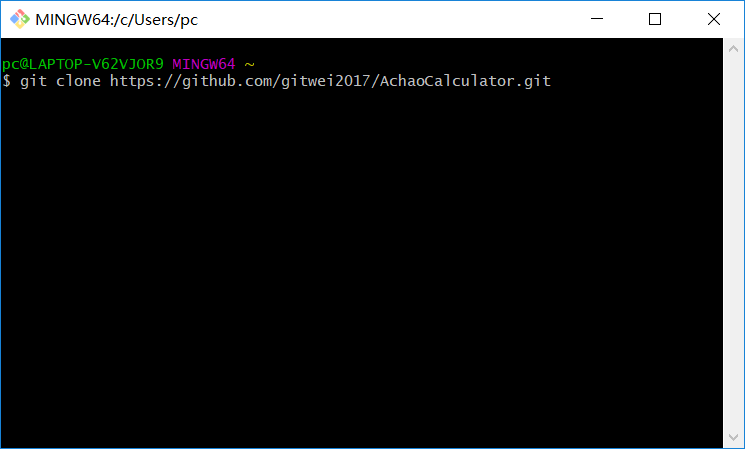# 代码设计

#include <iostream>
#include <stdlib.h>
#include <conio.h>
using namespace std;

void DealFenshu(int m, int a[])
{
for (int p = 0; p < m; p++)
{
int i = (int)rand() % 10;
int j = (int)rand() % 10;
while (j == 0 || i >= j)
{
i = (int)rand() % 10;
j = (int)rand() % 10;
}
int x = (int)rand() % 10;
int y = (int)rand() % 10;
while (y == 0 || x >= y)
{
x = (int)rand() % 10;
y = (int)rand() % 10;
}
int k = (int)rand() % 100 / 25;
switch (k)
{
case 0:
cout << "(" << i << "/" << j << ")" << "+" << "(" << x << "/" << y << ")" << "=";
a[p] = i * y + x * j;
a[p] = j * y;
break;
case 1:
cout << "(" << i << "/" << j << ")" << "-" << "(" << x << "/" << y << ")" << "=";
a[p] = i * y - x * j;
a[p] = j * y;
break;
case 2:
cout << "(" << i << "/" << j << ")" << "*" << "(" << x << "/" << y << ")" << "=";
a[p] = i * x;
a[p] = j * y;
break;
case 3:
a[p] = i * y;
a[p] = j * x;
cout << "(" << i << "/" << j << ")" << "/" << "(" << x << "/" << y << ")" << "=";
}

if (p % 5 == 4)
{
cout << endl;
}
else
{
cout << "\t";
}
}

}
void DisplayFenshu(int a[], int w, int m)
{
if (w == 1)
{
for (int q = 0; q < m; q++)
{
if (a[q] == 0)
cout << "0" << "\t";
else
cout << a[q] << "/" << a[q] << "\t";
if (q % 5 == 4)
{
cout << endl;
}
}
}
else
{
};
}
void DealInt(int m, int a[])
{

for (int p = 0; p < m; p++)
{
int i = (int)rand() % 10;
int j = (int)rand() % 10;
int k = (int)rand() % 100 / 25;
switch (k)
{
case 0:
cout << i << "+" << j << "=";
a[p] = i + j;
break;
case 1:
cout << i << "-" << j << "=";
a[p] = i - j;
break;
case 2:
cout << i << "*" << j << "=";
a[p] = i * j;
break;
case 3:
try
{
a[p] = i / j;
cout << i << "/" << j << "=";
}
catch (...)
{
p--;
}
}

if (p % 5 == 4)
{
cout << endl;
}
else
{
cout << "\t";
}
}
}
void DisplayInt(int a[], int w, int m)
{
if (w == 1)
{
for (int q = 0; q < m; q++)
{
cout << a[q] << "\t";
if (q % 5 == 4)
{
cout << endl;
}
}
}
else
{
};
}
void main()
{
int p;
do
{
system("cls");
int a, b;
int m, n, w;
cout << "请输入生成的四则运算题个数：";
cin >> m;
cout << endl;
cout << "请输入要生成的四则运算种类(输入1为整数，否则为真分数):";
cin >> n;
cout << endl;
if (n == 1)
{
DealInt(m, a);
cout << endl;
}
else
{
DealFenshu(m, b);
cout << endl;
}
cout << "是否输出答案(输入1则输出答案否则不输出答案)" << endl;
cin >> w;
if (n == 1)
{
DisplayInt(a, w, m);
}
else
{
DisplayFenshu(b, w, m);
}
cout << endl;
cout << "是否继续生成运算题(输入1则生成否则不生成)" << endl;
cin >> p;
cout << endl;
} while (1 == p);

}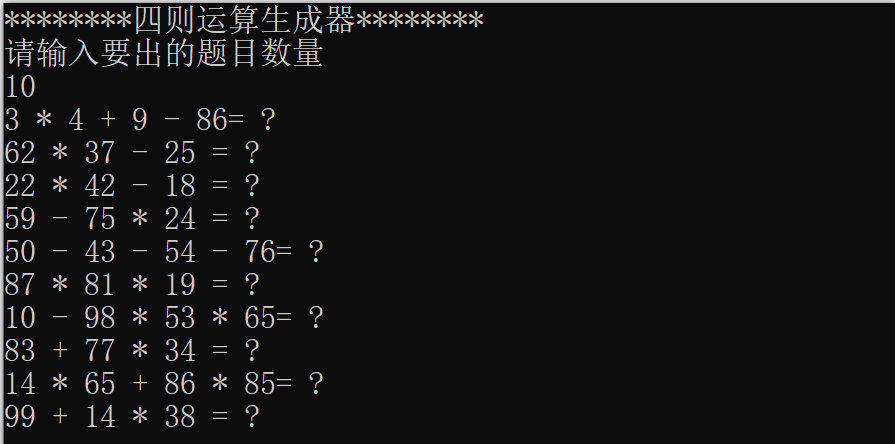# 克隆代码及提交代码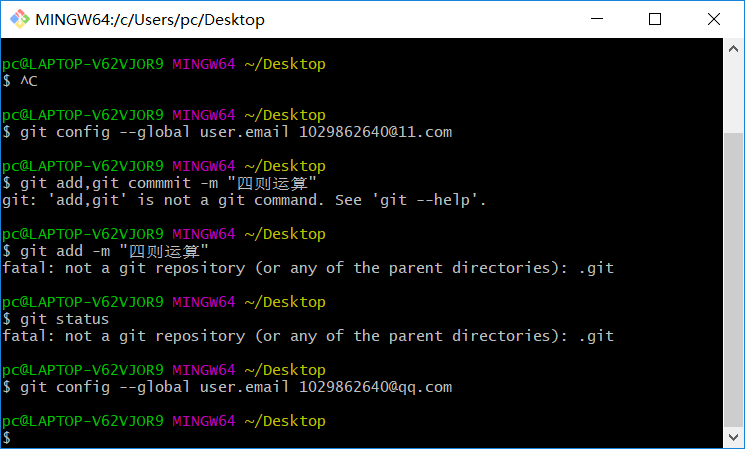# 总结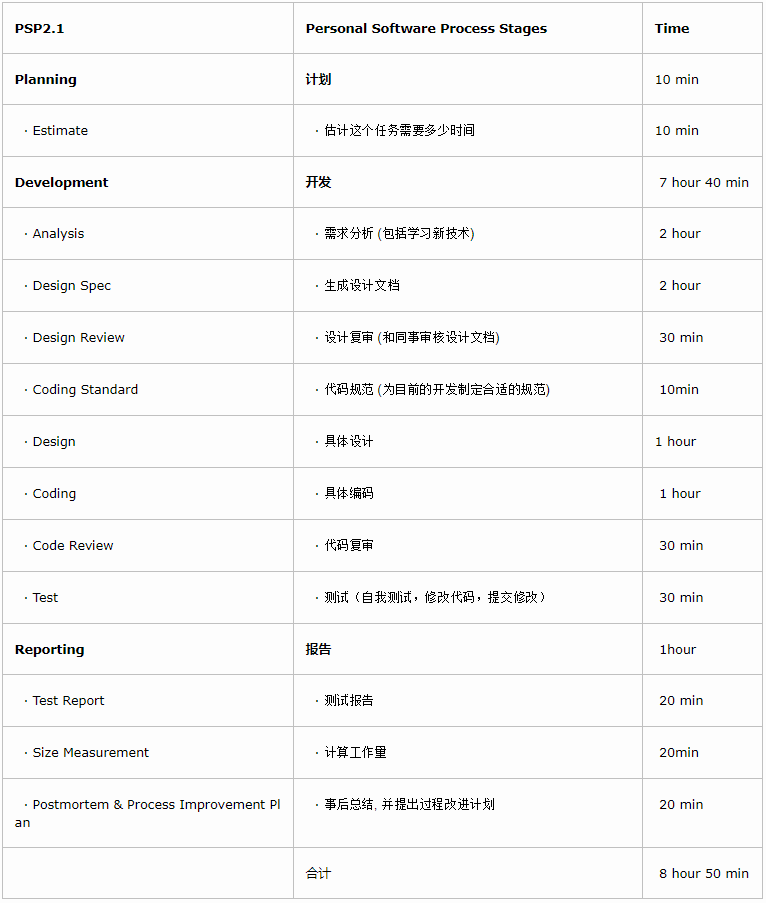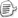posted on 2019-09-21 16:40  CharlieWhy  阅读(308)  评论(3编辑  收藏  举报